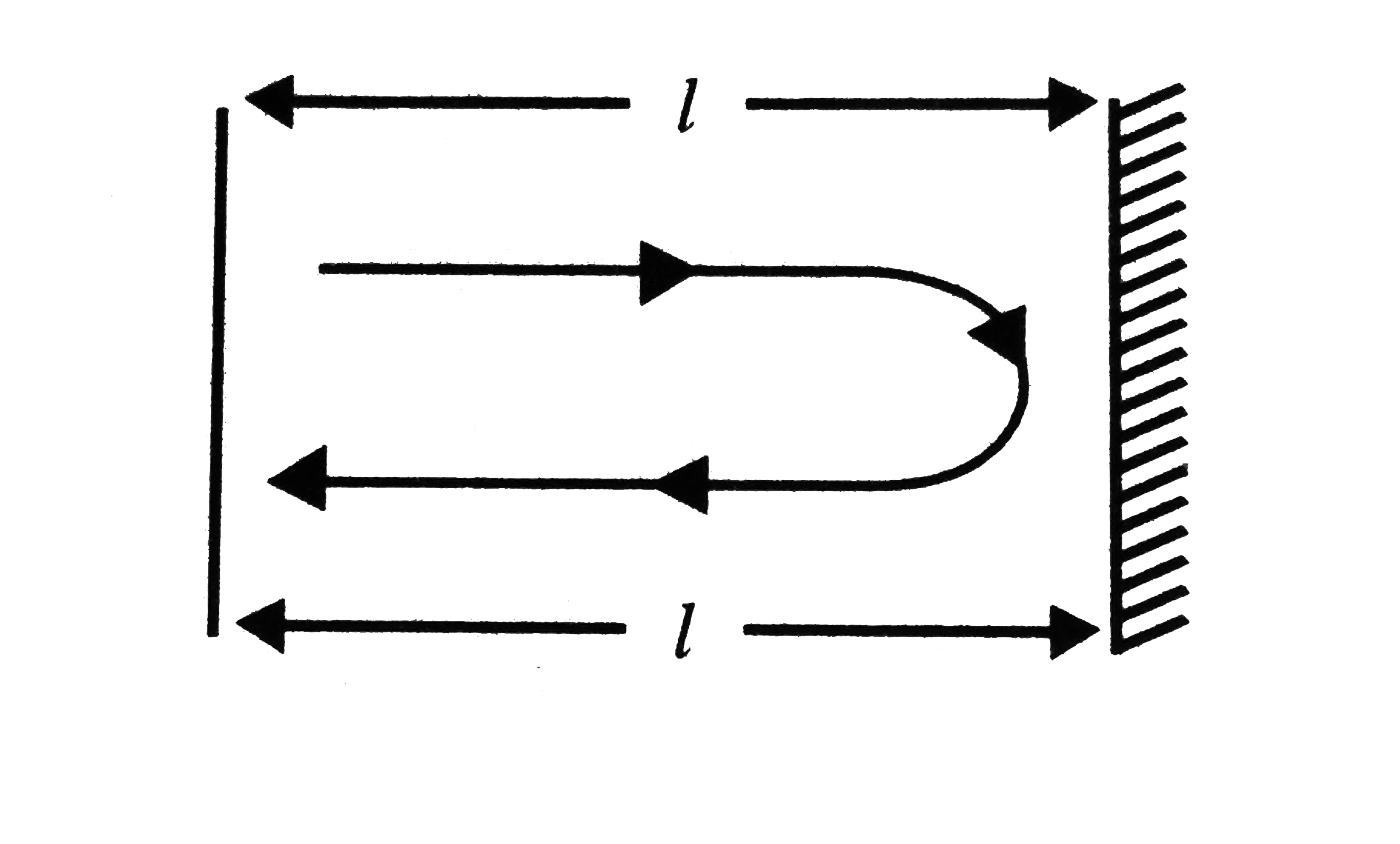# A man standing in front of a mountain beats a drum at regular intervals. The drumming rate is gradually increased and he finds that echo is not heard

49 views
in Physics
closed
A man standing in front of a mountain beats a drum at regular intervals. The drumming rate is gradually increased and he finds that echo is not heard distinctly when the rate becomes 40 per minute. He then moves near to the mountain by 90 metres and finds that echo is again not heard distinctly when the drumming rate becomes 60 per minute. Calculate (a) the distance between the mountain and the initial position of the man and (b) the velocity of sound.
A. i.330 m
ii. 330 m//s
B. i. 300 m
ii. 720 m//s
C. i. 240 m
ii. 300 m//s
D. i. 270 m
ii. 270 m//s

by (86.2k points)
selected

Drumming frequency  = 40 "cycle//min" = 40 "cycle//60s"
Drumming time period
T = (1)/(f) = ( 60 s)/( 40 "cycle") = (3)/(4) s//"cycle"
( time duration between consecutive drumming)
During this time interval , if sound goes to mountain and comes back then echo will not be heard distinctly.
(3)/(4) = ( 2 l)/( v) (i)
Now if he moves 90 m. This situation arises at  t = 60 "cycle//min",
T = (1)/(f) = 1 s//"cycle"
for this case sound goes to mountain and comes back after time T//2:
(1)/(2) = ( 2 ( l - 90))/( v) (ii)
Solving Eqs. (i) and (ii)
so , l = 270 m
v = 720 m//s`